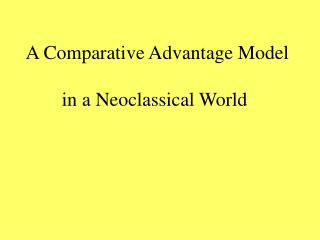# A Comparative Advantage Model - PowerPoint PPT PresentationDownload PresentationDownload Presentation- - - - - - - - - - - - - - - - - - - - - - - - - - - E N D - - - - - - - - - - - - - - - - - - - - - - - - - - -
##### Presentation Transcript

1. A Comparative Advantage Model in a Neoclassical World

2. A Two-Input Economy: A Neoclassical Model • A production function with two inputs: capital and labor • Capital and labor are imperfectly substitutable: an increasing cost production function • Isoquants • Isocosts • How does each firm decides on the mix of inputs: the profit maximizing rule

3. A production function: Q = f (K, L); given K, as L increases Q will increase; given L, as K increases Q will increase Capital, K , and Labor, L, are to some extent substitutable Both K and L are subject to decreasing returns. Special cases: Fixed-proportions production functions Perfectly substitutable inputs

4. An Isoquant: a graphical representation of a production function K Qx = f (K, L) Qx1 Qx0 L 0

5. The Isoquant: A Definition An isoquant is a curve drawn in a two-dimensional space representing all the combinations of two inputs (K and L), measured along the two axes, that can produce a given level of output. An isoquant map is a group of isoquants each representing a certain level of output for a given production function Isoquants farther from the origin represent higher levels of output Isoquants cannot cross

6. An Isoquant Map Qx = f (K, L) K Qx5 =100 Qx4 Qx3 Qx2 Qx1 Qx0=10 L 0

7. The slope of an isoquant: the marginal rate of technical substitution (MRTS) K Qx = f (K, L) Slope = ΔK/ΔL = MRTS A B Qx0 L 0

8. Production Costs: Isocost Given Q = f ( K, L ) Cost = C = r K + w L where r = price of K; w= price of L K C/r - w/r L o C/w

9. Equilibrium Qx = f (K, L) C = rK + wL K MRTS = w/r C/r a b Qx5 =100 Qx4 c Qx3 Qx2 Qx1 Qx0=10 L 0 C/w

10. K Equilibrium Qx = f (K, L) C = rK + wL Expansion Path MRTS = w/r C/r a b Qx5 =100 Qx4 c Qx3 Qx2 Qx1 Qx0=10 L 0 C/w

11. A Two-Industry Economy K (K/L)c Kc Computers (K/L)R W/r KR Rugs L o Lc LR

12. 0 K D L L Y X 0 K

13. A production possibilities curve for an Economy with two imperfectly substitutable resources producing two goods: Rugs MRS = MRT U1 Uo Computers o

14. Two Countries UA R UM R C C o o U.S. Mexico

15. A two-Country Model R R p C’ c f P’ F’ C C o o U.S. Mexico

16. Can these two countries gain from trade? Y Y Ua Ub 0 0 X X Country B Country A

17. Two Countries UA R R UB C C o o U.S. Mexico Can these two countries gain from trade?